# MP Board Class 12th Maths Solutions Chapter 2 Inverse Trigonometric Functions Ex 2.1

In this article, we share MP Board Class 12th Maths Book Solutions Chapter 2 Inverse Trigonometric Functions Ex 2.1 Pdf, These solutions are solved by subject experts from the latest MP Board books.

## MP Board Class 12th Maths Solutions Chapter 2 Inverse Trigonometric Functions Ex 2.1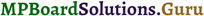Find the principal values of the following.

Question 1.
sin-1$$\frac { -1 }{ 2 }$$
Solution: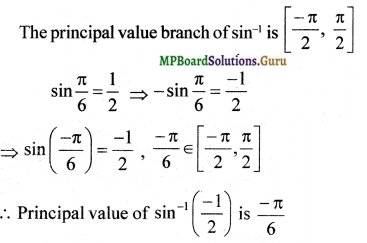Question 2.
cos-1$$\left(\frac{\sqrt{3}}{2}\right)$$
Solution: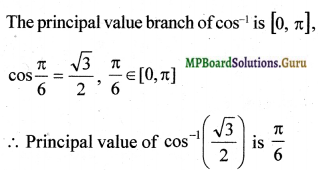Question 3.
cosec-1(2)
Solution:
The principal values branch of cosec-1 is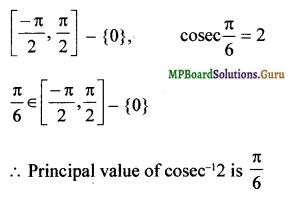Question 4.
tan-1(-$$\sqrt{3}$$)
Solution:
The principal values branch of tan-1 is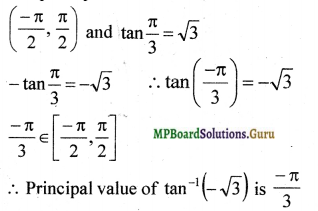Question 5.
cos-1$$\frac { – 1 }{ 2 }$$
Solution:
The principal values branch of cos-1 is [0, π]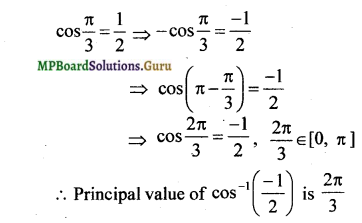Question 6.
tan-1(-1)
Solution:
The principal values branch of tan-1 is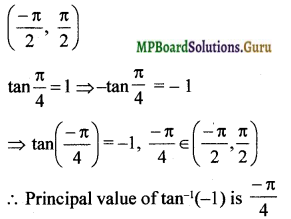Question 7.
sec-1$$\left(\frac{2}{\sqrt{3}}\right)$$
Solution:
The principal values branch of sec-1 is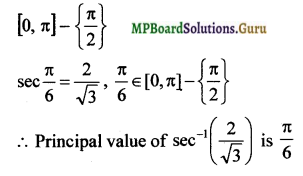Question 8.
cot-1($$\sqrt{3}$$)
Solution:
The principal values branch of cot-1 is [0, π]
cot$$\frac { π }{ 6 }$$ = $$\sqrt{3}$$, $$\frac { π }{ 6 }$$ ∈ [0, π]
∴ Priciapal value of cot-1$$\sqrt{3}$$ is $$\frac { π }{ 6 }$$

Question 9.
cos-1$$\left(\frac{-1}{\sqrt{2}}\right)$$
Solution:
The principal values branch of cos-1 is [0, π]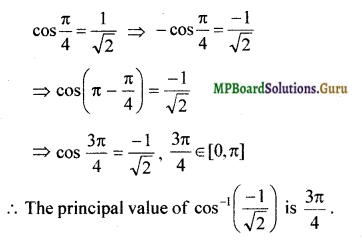Question 10.
cosec-1($$\sqrt{-2}$$)
Solution:
The principal values branch of cosec-1 is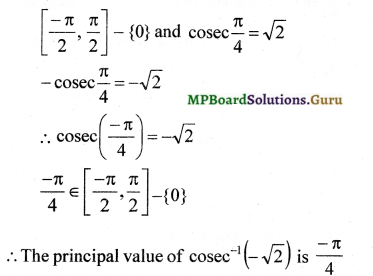Question 11.
tan-1(1) + cos-1($$\frac { – 1 }{ 2 }$$) + sin-1($$\frac { – 1 }{ 2 }$$)
Solution: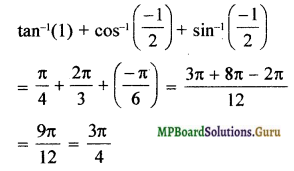Question 12.
cos-1($$\frac { 1 }{ 2 }$$) + 2 sin-1($$\frac { 1 }{ 2 }$$)
Solution: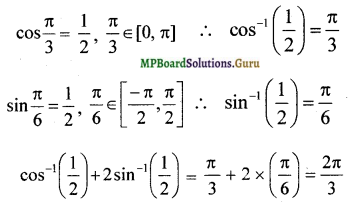Question 13.
If sin-1 x = y, then
a. 0 ≤ y ≤ π
b. – $$\frac { π }{ 2 }$$ ≤ y ≤ $$\frac { π }{ 2 }$$
c. 0 < y < π
d. – $$\frac { π }{ 2 }$$ < y < $$\frac { π }{ 2 }$$
Solution:
b. – $$\frac { π }{ 2 }$$ ≤ y ≤ $$\frac { π }{ 2 }$$
The principal values branch of sin-1 is
[$$\frac { – π }{ 2 }$$, $$\frac { π }{ 2 }$$] . i.e., $$\frac { – π }{ 2 }$$ ≤ y ≤ $$\frac { π }{ 2 }$$Question 14.
tan-1$$\sqrt{3}$$ – sec-1 ( – 2) is equal to
a. π
b . $$\frac { – π }{ 3 }$$
c. $$\frac { π }{ 3 }$$
d. $$\frac { 2π }{ 3 }$$
Solution:
b . $$\frac { – π }{ 3 }$$
tan-1$$\sqrt{3}$$ – sec-1 ( – 2) = $$\frac { π }{ 3 }$$ – $$\frac { 2π }{ 3 }$$ = $$\frac { – π }{ 3 }$$